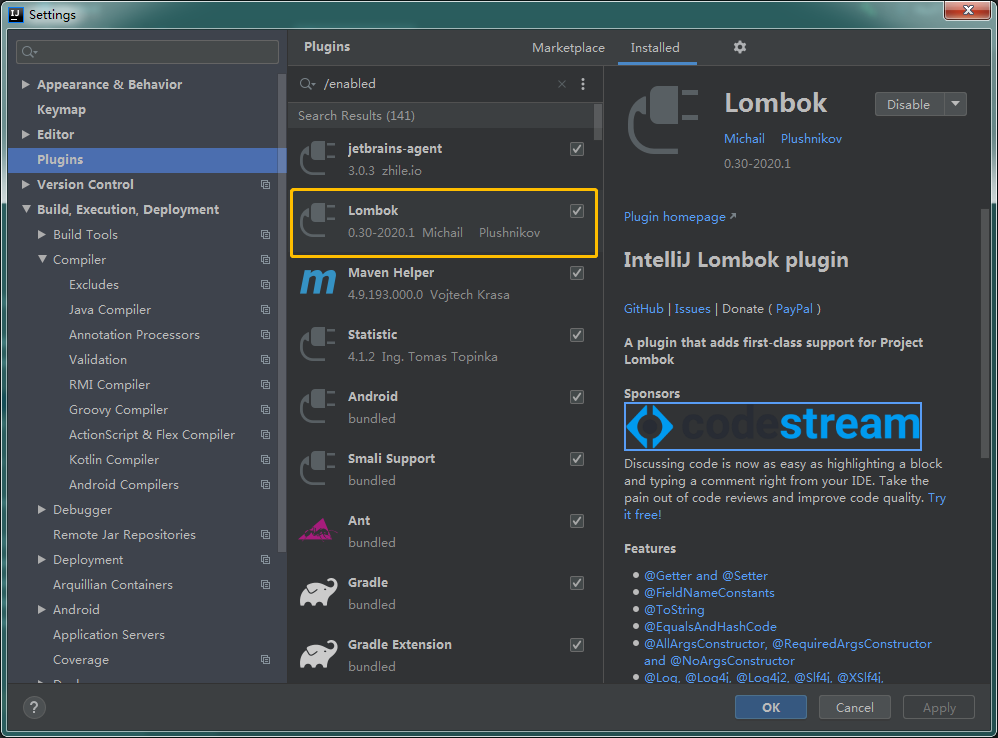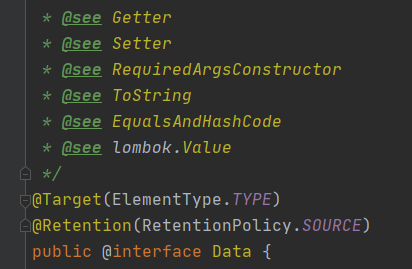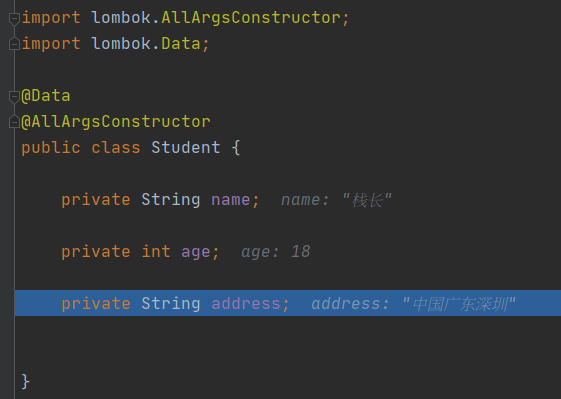## Lombok 的弊端

Lombok 的好处就不说了，就是帮我们大量简化代码，这里重点说下为什么有人不推荐使用 Lombok

#### 一、需要额外的组件

1）Lombok 依赖包

<dependency>
<groupId>org.projectlombok</groupId>
<artifactId>lombok</artifactId>
<version>1.18.12</version>
<scope>provided</scope>
</dependency>


2）Lombok IDE 插件

Eclipse/ IntelliJ IDEA 都提供了 Lombok 插件，用来识别 Lombok 的注解，否则会显示编译报错。如果你对 IDEA 还不会使用可以关注Java技术栈公众号在后台回复IDEA获取系列教程。

IntelliJ IDEA 插件示例：#### 二、@Data 注解的坑

@Data 注解用在类上，等同于下面这几个注解合集：• @Getter
• @Setter
• @RequiredArgsConstructor
• @ToString
• @EqualsAndHashCode

@Getter, @Setter, @ToString 很简单，用起来也没问题，而 @RequiredArgsConstructor@EqualsAndHashCode 需要注意下。

1）RequiredArgsConstructor

Generates a constructor with required arguments.Required arguments are final fields and fields with constraints such as {@code @NonNull}.

2）@EqualsAndHashCode

Generates implementations for the {@code equals} and {@code hashCode} methods inherited by all objects, based on relevant fields.

/**
* Call on the superclass's implementations of {@code equals} and {@code hashCode} before calculating for the fields in this class.
* <strong>default: false</strong>
*
* @return Whether to call the superclass's {@code equals} implementation as part of the generated equals algorithm.
*/
boolean callSuper() default false;


@Data
public class BaseStudent {

private int id;

}

@Data
@AllArgsConstructor
public class Student extends BaseStudent {

private String name;

private int age;

}


public boolean equals(Object o) {
if (o == this)
return true;
if (!(o instanceof Student))
return false;
Student other = (Student)o;
if (!other.canEqual(this))
return false;
Object this$name = getName(), other$name = other.getName();
if ((this$name == null) ? (other$name != null) : !this$name.equals(other$name))
return false;
if (getAge() != other.getAge())
return false;
Object this$address = getAddress(), other$address = other.getAddress();
return !((this$address == null) ? (other$address != null) : !this$address.equals(other$address));
}

public int hashCode() {
int PRIME = 59;
result = 1;
Object $name = getName(); result = result * 59 + (($name == null) ? 43 : $name.hashCode()); result = result * 59 + getAge(); Object$address = getAddress();
return result * 59 + (($address == null) ? 43 :$address.hashCode());
}


#### 三、代码跟踪调试## 总结

Lombok 虽好，你也要遵循团队的规范，能用的情况下再用，也不能乱用，不了解其构造，乱用就容易出现问题的。